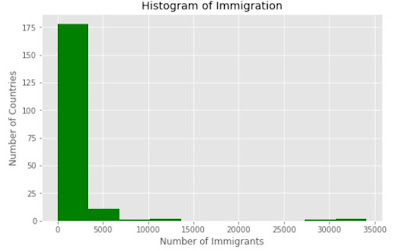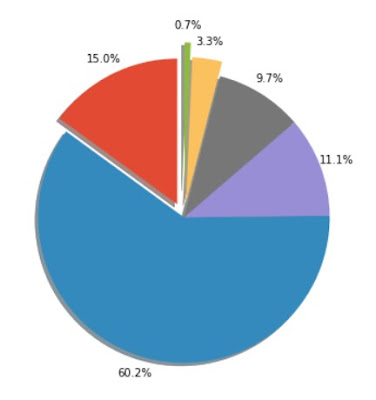### Visualization of Data with Python (Matplotlib and Seaborn)

Data Visualization is key to visualize and understand what the relationship is there between the data given to us. It also helps us to convey our findings to the stake holders in a very presentable and understandable manner.

We basically use two packages Matplotlib and Seaborn for plotting of figures in python.

### Lets see the plots we can make in python:

import matplotlib.pyplot as plt
import seaborn as sns

## 1. Line plot

A line plot or or line chart is a type of plot which displays information as a series of data points called 'markers' connected by straight line segments. Line plot should be used when we have continuous data.Line Plots

## 2. Area Plots

An area plot is similar to line plot, except that the area between the x-axis and the line is filled with color or shading.  It represents the evolution of a numerical variable following another numerical variable.Area Plots

## 3. Histogram Plots

A histogram is the most commonly used graph to show frequency distribution.Histogram Plots

## 4. Bar Plots

A bar plot is a chart that represents categorical data with rectangular bars with heights or lengths proportional to the values that they represent. The bars can be plotted horizontally or vertically.Bar Plots

## 5. Pie Chart

A pie chart is a circular graphic that displays numeric proportions by dividing  a circle (pie) into proportional slices.Pie Chart

## 6. Box Plots

A box plot is a way of statistically representing the distribution of the data through five main parameters and they are minimum - smallest number in the data set, first quartile, Median, third quartile and maximum - highest number in the data set. There are outliers also.Box plots

The other plots are scatter plots, bubble plots, Waffle charts, regression plot, residual plot, distribution plot and many more.